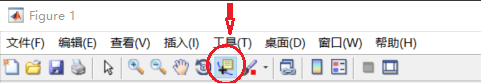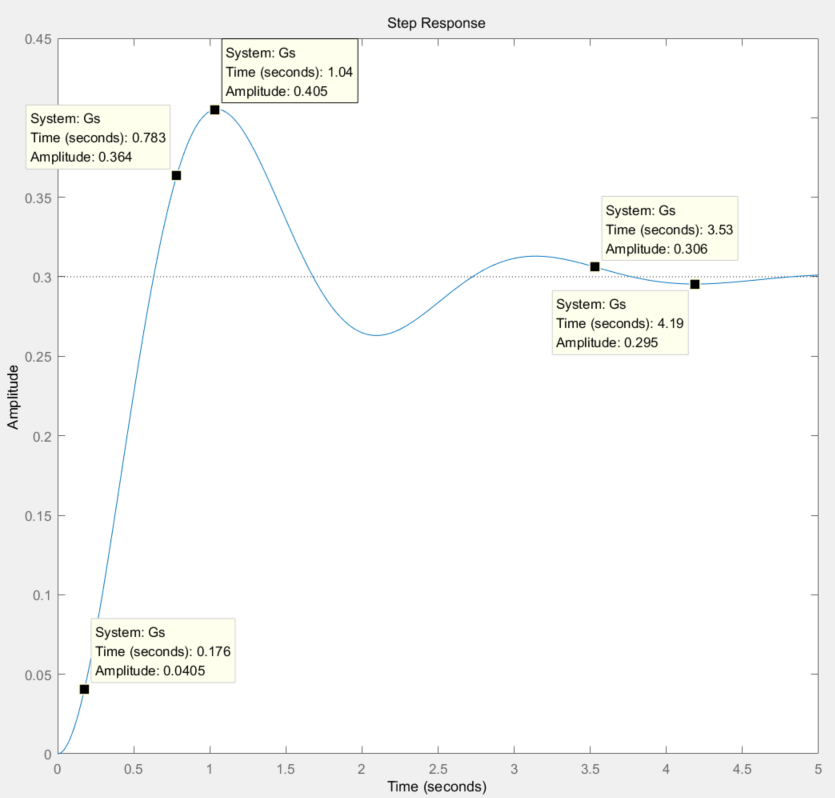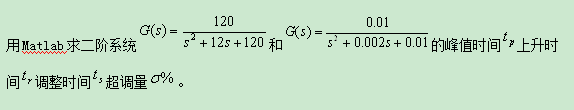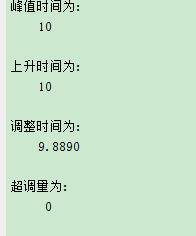• 上升时间 tr：响应从峰值的10%上升到峰值的90%所需要的时间；而阶跃响应则是从终值的10%上升到终值的90%所需要的时间；对有振荡的系统，也可以定义为从0到第一次到达终值所需的时间。 峰值时间 tp：响应越过终值...
1. 准备
终值：c(∞) 上升时间 tr：响应从峰值的10%上升到峰值的90%所需要的时间；而阶跃响应则是从终值的10%上升到终值的90%所需要的时间；对有振荡的系统，也可以定义为从0到第一次到达终值所需的时间。 峰值时间 tp：响应到达第一个峰值所需的时间。 调节时间 ts：响应到达并保持在终值的正负5%误差带内所需的最短时间；有时也用终值的正负2%。 超调量 σ%：峰值c(tp )超出终值的百分比，即：题目：
已知二阶系统传递函数为试分别用游动鼠标法和编程法求取系统的峰值，超调量，上升时间以及调节时间。
2. 编程法：
s = tf('s');   % 用字母 s 构造传递函数
Gs = 3/(s^2+2*s+10); % 构造 Gs
t = 0:0.005:5; % 设置横轴范围和步长
[y,x,t] = step(Gs,t); % 根据步长逐步响应传递函数
[ymax,tp] = max(y); % 获取最大值的点的数据

r=1;
r1=0;
while y(r) < (ymax*0.9) % 过滤小于90%的
if y(r) > (ymax*0.1) % 过滤大于10%的
r1=r1+1;
end;
r=r+1;
end;

rise_time = r1*0.005 % 上升时间
peak_time = (tp-1)*0.005 % 峰值时间
ystable = dcgain(Gs); % 稳态值
max_overshoot = (ymax-ystable)/ystable % 超调量

r2=1001; % 由 (5-0)/0.005+1 求得
while y(r2) > ystable*0.98 && y(r2) < ystable*1.02
% 从稳态倒推回去，求得首次达到并维持在稳态值正负2%的范围的值
r2 = r2 - 1;
end
settle_time = (r2-1)*0.005 % 调节时间

求得：
rise_time =   0.6100
peak_time = 1.0450
max_overshoot = 0.3509
settle_time = 3.5350

3. 游动鼠标法：
游动鼠标法不适合用于plot()命令画出的图形，只能在非plot()函数输出的曲线上求取。 1.获取必要数据
s = tf('s');
Gs = 3/(s^2+2*s+10); % 构造 Gs
t = 0:0.005:5;
step(Gs,t)  % 画出图像
[y,x,t] = step(Gs,t);
[ymax,tp] = max(y);
ymax	% 峰值
ym10 = ymax * 0.1  % 峰值 10%
ym90 = ymax * 0.9  % 峰值 90%
ystable = dcgain(Gs) % 稳态值
ys98 = ystable * 0.98  % 稳态 -2%
ys102 = ystable * 1.02   %  稳态 +2%

得到图像并求得：
ymax = 0.4053
ym10 = 0.0405
ym90 = 0.3647
ystable = 0.3000
ys98 = 0.2940
ys102 = 0.3060

2.点击工具栏中的数据游标，在图中分别标出纵坐标为 ymax = 0.4053，ym10 = 0.0405，ym90 = 0.3647，ys98 = 0.2940，ys102 = 0.3060 的五点：3.收集图像中 Time 对应的 Amplitude 数据，并计算可得： tr = 0.783 - 0.176 = 0.607 tp = 1.04 ts = 3.53 (因为0.295位于此波谷最低处，而0.295>0.294，故不取4.19) σ% = (0.405 - 0.3)/0.3 × 100% = 35%
下面是另外两个博客，和这个关系比较密切，关于 PID 算法解释的： PID算法模型分析：基于温度控制 临界比例度法整定P、PI、PID控制器的参数的matlab算法实现
>>> End <<<
展开全文超调量 峰值时间
• (上升时间、调整时间、峰值峰值、超调量概述阶跃响应性能指标定义Matlab函数示例求一阶系统阶跃响应性能指标求复杂系统阶跃响应性能指标求解不同阻尼比时二阶系统的阶跃响应性能指标联系作者 (上升时间、调整时间、...


Matlab求解阶跃响应性能指标
概述阶跃响应性能指标定义Matlab函数示例求一阶系统阶跃响应性能指标求复杂系统阶跃响应性能指标求解不同阻尼比时二阶系统的阶跃响应性能指标
联系作者

概述
工科的同学对阶跃响应应该不会陌生，简单来说，阶跃响应是指输入量发生阶跃变化时动态系统的输出阶跃响应，通过分析输出阶跃响应的性能指标，可以分析和比较动态系统的动态性能和稳态性能。
阶跃响应性能指标定义
阶跃响应性能指标主要包括稳态值、上升时间、峰值时间和超调量，定义如下： 稳态值ys：当时间趋近于无穷大时，阶跃响应的输出值，ys=y(∞)。 上升时间tr：输出阶跃响应达到90%稳态值时所对应的时刻。 峰值时间tm：输出阶跃响应峰值ym所对应的时刻。 超调量σ：输出阶跃响应峰值ym与稳态值ys之差所占稳态值ys的百分比, σ%= (ym-ys)/ys。 调整时间ts：输出阶跃响应进入稳态值ys±Δ误差带范围内所对应的时刻, 一般取Δ=0.02或Δ=0.05。Matlab函数
按照阶跃响应性能指标的定义，作者使用Matlab开发了函数Fun_Step_Performance.m，使用数值算法求出各类阶跃响应的性能指标值，函数简单、易用、通用性好。
function [ys,tr,ts,tm,ov] = Fun_Step_Performance(t,y,drawflag)
% [ys,tr,ts,ov] = Fun_Step_Performance(t,y) 标准阶跃响应的性能指标求解
% 本程序适用于标准阶跃响应曲线，末尾时间必须已经接近稳态值
% t-y 为阶跃响应的时间-输出配对序列，可由[y,t] = step(sys)求得
% drawflag为时候作图标志，不输入或输入非0值时，默认作图，输入0时不做图
% ys 稳态值
% tr 上升时间，默认为0-90%的上升时间
% ts 调整时间，默认为2%的调整时间
% tm 为峰值时间
% ov 超调量 %
% e.g.
%  sys = tf(1,[1 2*0.5*1 1]);
%  [y,t] = step(sys,15);
%  [ys,tr,ts,tm,ov] = Fun_Step_Performance(t,y,1);

示例
求一阶系统阶跃响应性能指标
% Eg 1 一阶系统
sys = tf(1,[3 1]);
[y,t] = step(sys,25);
[ys,tr,ts,tm,ov] = Fun_Step_Performance(t,y);%% 阶跃响应指标结果：
上升时间：7s
调整时间：11.5s
峰值时间：25s，超调量：0%
稳态值：1
%% 阶跃响应指标结果显示结束

求复杂系统阶跃响应性能指标
sys = tf(1,[1 2*0.20*1 1]) * tf(1,[2 1]) * tf([1.5 1],[1 2*0.25*3 9]);  % 5阶系统
[y,t] = step(sys,35);
[ys,tr,ts,tm,ov] = Fun_Step_Performance(t,y);%% 阶跃响应指标结果：
上升时间：2.0877s
调整时间：17.3158s
峰值时间：3.5614s，超调量：40.1285%
稳态值：0.111
%% 阶跃响应指标结果显示结束

求解不同阻尼比时二阶系统的阶跃响应性能指标
% Eg 3 求解不同阻尼比时二阶系统的阶跃响应特性
wn = 1;    % 固有频率
kes_vet = [0.2 0.4 0.6 0.8 1 1.2];   % 阻尼比序列
figure
hold on
for ii = 1:length(kes_vet)
kes = kes_vet(ii);
sys = tf(1,[1 2*kes*wn wn^2]);   % 二阶系统传递函数
[y,t] = step(sys,50);            % 阶跃响应
[ys(ii),tr(ii),ts(ii),tm(ii),ov(ii)] = Fun_Step_Performance(t,y,0);  % 求解阶跃响应,不绘图
plot(t,y)
Str{ii} = [ '\xi = '  num2str(kes)];
end
legend(Str)
xlabel('时间t/s')
ylabel('输出响应y')

figure
subplot(221)
plot( kes_vet,tr,'-o' )
xlabel('阻尼比\xi')
ylabel('上升时间/s')
subplot(222)
plot( kes_vet,ts,'-o' )
xlabel('阻尼比\xi')
ylabel('调整时间/s')
subplot(223)
plot( kes_vet,tm,'-o' )
xlabel('阻尼比\xi')
ylabel('峰值时间/s')
subplot(224)
plot( kes_vet,ov,'-o' )
xlabel('阻尼比\xi')
ylabel('超调量/%')tr =
1.8349    2.0737    2.4540    2.9954    4.0000    4.9407

ts =
19.2661    8.2949    5.8282    3.6866    5.5000    7.9051

tm =
3.2110    3.4562    3.9877    5.1843   40.0000   50.0000

ov =
52.6622   25.3725    9.4610    1.5144         0         0

对于二阶系统，阻尼比的变化不影响输出稳态值，随着阻尼比增加，上升时间逐步增大、调整时间先减小再增大、峰值时间逐步变大、超调量逐步变小；当阻尼比在0.707左右时，上升时间和调整时间较快，且超调量很小，系统综合性能较好，工程上通常设计阻尼比在0.707左右，称之为最佳阻尼比。
联系作者
源程序下载:
https://mp.weixin.qq.com/s?__biz=MzAwOTk1NDcyMw==&mid=2247483741&idx=1&sn=b37d10b7ae5f5feee6a967ec77682991&chksm=9b56f591ac217c87040918b60b053d3c95a93476509502c643f4151b96140c91aa346262c846&token=1240415358&lang=zh_CN#rd扫码关注微信公众号Matlab Fans，回复BK06获取百度网盘下载链接。展开全文matlab simulink 控制器
• 这是一个函数，它依次返回系统的延迟时间、上升时间、峰值时间、最大过冲、稳态误差稳定性的值。matlab
• 原创开发，使用Matlab求解阶跃响应指标(上升时间、调整时间、峰值峰值、超调量)，内含自定义Matlab函数、丰富的演示实例详细的说明文档，可以方便求取系统阶跃响应指标，简单易用。
• 以下是第二个传递函数的做法，若想求第一个或其他的传递函数的参数，只需要改变分子矩阵num分母矩阵即可 num1=[0.01]; den1=[1,0.002,0.01]; t=0:0.001:10;%为了确保精度，即各个参数的小数的位数，减小步长 y=...以下是第二个传递函数的做法，若想求第一个或其他的传递函数的参数，只需要改变分子矩阵num和分母矩阵即可
num1=[0.01];
den1=[1,0.002,0.01];
t=0:0.001:10;%为了确保精度，即各个参数的小数的位数，减小步长
y=step(num1,den1,t);
n=length(t);
[ymax,ind]=max(y);%y是原系统的阶跃响应，是一个二维矩阵，返回本身曲线的最大值和对应的时间矩阵变量的第几位
yss=y(n);%我们这里就设我们时间的最终值对应的就是稳态值
mp=(ymax-yss)/yss;%由超调量定义可以计算超调量
for k=1:n%该层循环是计算上升时间即第一次达到稳态值的时间
if y(k)<=yss&y(k+1)>=yss%第一次达到稳态值
tr=t(k+1);%用tr存储稳态值
break
end
end
for i=n:-1:1%该层循环用于计算调整时间，我们是从最终时间（无穷远的时间）往前开始遍历查找，否则从前开始查找的话会出错
if y(i)>=1.02*yss|y(i)<=0.98*yss%当得到的值与稳态值差的绝对值与稳态值之比为2%时说明到达了调整时间
ts=t(i);%ts存储调整时间
break
end
end
disp('峰值时间为:')
disp(t(ind))
disp('上升时间为:')
disp(tr)
disp('调整时间为:')
disp(ts)
disp('超调量为:')
disp(mp)展开全文• 2.用Matlab求上升时间、峰值时间、最大过调量调整时间 定义闭环系统传递函数如下： H(s)=25/(s^2+6*s+25) %求上升时间、峰值时间、最大过调量调整时间 %可以应用到高阶系统 num=[0 0 25]; ...
1.Matlab作单位阶跃响应曲线的三维图

定义闭环系统传递函数如下：
H(s)=1/(s^2+2*zeta*s+1)

%Matlab作单位阶跃响应曲线的三维图
%标准二阶系统响应曲线 wn=1
t=0:0.2:10;
zeta=[0 0.2 0.4 0.8 1];
for n=1:6
num=1;
den=[1 2*zeta(n) 1];
[y(1:51,n),x,t]=step(num,den,t) ;
end

plot(t,y)

mesh(t,zeta,y')

2.用Matlab求上升时间、峰值时间、最大过调量和调整时间
定义闭环系统传递函数如下：
H(s)=25/(s^2+6*s+25)

%求上升时间、峰值时间、最大过调量和调整时间
%可以应用到高阶系统
num=[0 0 25];
den=[1 6 25];
t=0:0.005:5;
[y,x,t]=step(num,den,t) ;
r=1;
while y(r)<1.0001;r=r+1;end;
rise_time=(r-1)*0.005

[ymax,tp]=max(y);
peak_time=(tp-1)*0.005

max_overshoot=ymax-1

s=1001;while y(s)>0.98&y(s)<1.02;s=s-1;end;
settle_time=(s-1)*0.005

运行后结果：
rise_time =    0.5550
peak_time =   0.7850
max_overshoot =    0.0948
settle_time =    1.1850

求单位脉冲响应的两种方法（求斜坡响应也可用此方法）

在初始条件为零时，H(s)的单位脉冲响应也就是sH(s)的单位阶跃响应
H(s)=1/（s^2+0.2*s+1）

num=;
den=[1 0.2 1]；
impulse(num,den)

或

num=[0 1 0];
den=[1 0.2 1]；
step(num,den)
展开全文• 之前看到过一篇介绍已知传递函数分析系统动态特性静态特性的博客，但那个代码只能分析标准形式的传递函数，最终响应值为1，然而，如果传递函数化为标准形式之后分子上还有常系数，则其最终响应值不为1，用原代码...
• 在400KHz设置下，最小上升时间规范为300ns，在100KHZ系统中最小上升时间规范为1000nsc
• 理想脉冲信号总是理想的方波，但实际的物理系统由于器件特性耦合电容的存在，总会存在上升沿下降沿。因此，仿真中有时需要考虑这种特性的影响。本文的目的是实现给理想方波加上可调的上升沿下降沿。 1. 理想...
• 通过对这些时间序列的分析，从中发现揭示现象发展变化的规律，并将这些知识信息用于预测。比如销售量是上升还是下降，销售量是否与季节有关，是否可以通过现有的数据预测未来一年的销售额是多少等。 对于时间...python 数据分析
• ## 时间序列

千次阅读 2018-09-02 00:33:43
时间序列的定义 所谓时间序列就是按照时间的顺序记录的一列有序数据。对时间序列进行观察、研究、找寻他发展变化的规律，预测他将来的走势就是...协方差函数相关系数度量的是两个不同的事件彼此之间的相互影响...
• 时间继电器是一种将电子定时电路与继电器组合在一起的延时控制装置，在电子设备的自动控制中使用较多，通过它可以定时控制电路的换接。时司继电器具有使用方便、定时可调、转换触点多及使用寿命长的特点，但其外形...
• matlab开发-传递函数的时间响应参数不同。获取延迟时间、上升时间、峰值时间、最大超调量、稳态误差稳定性的值
• 这个项目被称为water-clock：是在头脑风暴阶段的... 如果有一个计数器，数字将上升或下降，如果是时钟，则显示的时间后面可能会有一些意义。 工具技术： 计时器，时区，javascript时间方法数据库？，身份验证？JavaScript
• 本文转载自IT之家，下周日，美国又要进入夏令时模式。届时硅谷北京的时差将从 16 个小时变成 15 个小时。过去半个世纪，美国人每年春秋都要调整两次时间。...美国中国国土面积相近，但中国统一使用北京时间不同，
• ## 算法模型---时间序列模型

万次阅读 多人点赞 2018-01-16 09:04:58
1、时间序列时间序列是时间间隔不变的情况下收集的不同时间点数据集合，这些集合被分析用来了解长期发展趋势及为了预测未来。...常用的时间序列模型有AR模型、MA模型、ARMA模型ARIMA模型等。2、时间
• 时间依存协变量，以及一些常见错误； 制作时间依存协变量的工具，更确切的说是如何编码相关数据集； 时间依存系数。 2 Time dependent covariates One of the strengths of the Cox model is its ability to ...算法 r语言
• 根据呼吸器减压阀传递函数模型,利用Matlab软件对减压阀整个工作过程进行了数值仿真,运用最大超调量、上升时间和调节时间3个时域指标判定减压阀响应特性,得到了单一结构参数和工作参数对减压阀出口压力动态响应曲线,...
• 图所示为线性时不变自动调节系统的典型阶跃响应曲线y（u），其输人为单位阶跃函数I（t），...　（2）上升时间tr，输出响应从稳态值的10％上升到90％所需的时间。 　（3）峰值时间tp，输出响应到达第一个峰值所需的时
• 时间序列异常检测--简单的语言介绍当前时间序列异常检测方法 在Statsbot，我们不断地回顾异常检测方法，并在此基础上改进我们的模型。 本文概述了目前最流行的时间序列异常检测算法及其优缺点。 这篇文章是写给...
• 使用CUBE_MX生成带有free_RTOS操作系统的工程，我们经常会使用到系统相对时间，尤其是使用其做数据采集的项目中经常需要给你数据打上时间的标签就需要实时获取系统的相对时间。 当然，我们可以单独开一个时钟来计时...stm32 CUBE
• ## python时间序列数据预测教程之 arima

千次阅读 热门讨论 2020-04-14 23:27:01python
• （十一）时间序列分析预测 时间序列数据用于描述现象随时间发展变化的特征。 时间序列（times series）是同一现象在不同时间的相继观察值排列而形成的序列。经济数据大多数以时间序列的形式给出。 时间序列及其...
• 本设计实例介绍了一种基于OVP/UVP测试、负载余量测试、电压可编程性或其它任何理由而需要调节电源输出电压的方法。图1电源输出电压双向调节电路。图1所示电路可以通过向反馈节点输出或输入电流实现...由U5U6组成的
• 冒泡排序、插入排序、归并排序基数排序是稳定的排序算法。 冒泡法： 这是最原始，也是众所周知的最慢的算法了。他的名字的由来因为它的工作看来象是冒泡：复杂度为O(n*n)。当数据为正序，将不会有交换。...排序算法 排序稳定性 排序算法分析
• 文章目录动态性能介绍上升时间trt_{r}tr​峰值时间tpt_{p}tp​调节时间tst_{s}ts​超调量σ%\sigma\%σ%小结稳态性能介绍线性系统的稳定性分析1. 稳定性的概念定义2. 稳定系统的充要条件3. 稳定判据（代数判据）(1...自动控制原理 笔记...